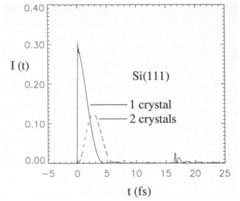# Dynamical diffraction of ultrashort X-ray free-electron laser pulses

## S.D. Shastri, P. Zambianchi & D.M. Mills, J. Synchrotron Rad. (2001). 8, 1131–1135

Nature can be tough with humans. It tells us that there are limits. For example, if we want to measure the energy of a particle (or a wave) very accurately we cannot do this in an 'ultrashort' time. Conversely, if we use a very short radiation pulse of length Δt to determine its energy there will be a 'natural' error bar ΔE given by the well-known uncertainty relation ΔEΔt ≥ 2.352h/4π where h is Planck’s constant. (The factor 2.352 takes care of the fact that we consider the full width at half maximum of Gaussian distribution functions.) This is precisely what the paper is about: it describes how a crystal would respond to a hypothetical pulse of infinitely short duration. Because the crystal is perfect, the diffraction process is starting from results of dynamical theory obtained for the angular or energy response of a perfect crystal in the steady-state plane-wave approximation. The Darwin–Prins curve is the input for the subsequent calculations of kinematical type using a 'continuous superposition' of Green’s functions. The result is, as can be expected, a temporal smearing of the incident delta function. Numerical results were calculated for the 111 and 444 reflections from silicon in the symmetrical Bragg case. Does this semidynamical approach give physically reasonable results?Delta-function-induced transient reflected intensity at 8 keV from one and two Si(111) Bragg crystals of thickness 10 μm.
There is another way of looking at the problem. For a given X-ray wavelength the beam has to penetrate a certain depth into the crystal to be fully reflected and to achieve the nominal monochromaticity of the reflection used. Although this penetration or extinction depth text (Io/e-depth perpendicular to the netplanes, Io is the incident intensity) varies as the crystal is swept across the Bragg angle, its average value can be formally related to the relative spectral resolution ΔE/E = d/text = 1/N where d is the spacing between the lattice planes and N the number of planes contributing to the reflection. The time delay Δt times c (the speed of light) per lattice plane is just the X-ray wavelength (Bragg’s law). Then the total delay between the part of the incoming intensity reflected from the surface and the one coming from an extinction depth below the surface is N times the wavelength. From this we get ΔEΔt = h, which agrees to within a factor of 2.5 with the relation given earlier. Inserting numbers: for 8 keV and the silicon 111 reflection (ΔE/E = 1.35 x 10–4) we get a time delay of 2.8 femtoseconds (fs) for the Io/2 depth. This agrees pretty well with the value of 2.0 fs from the impulse response calculations shown in the figure. Therefore, the two results from dynamical theory interpreted in a kinematical fashion are consistent and appear to be physically reasonable. It is important to note that a full dynamical approach of time-dependent diffraction by perfect crystals starting with the basic equations from Zachariasen (1945) gave a very similar result: 1.6 fs for 12.4 keV corresponding to 2.5 fs for 8 keV radiation (Sheppard et al., 2001). The result for a double nondispersive reflection is also shown in the figure and one can distinguish an echo coming from the back of the 10 μm thick crystal too. The shape is easy to understand: the intensity gradually decreases as the beam penetrates into the crystal and hence the part of the radiation coming later is less intense.

The theory was then applied to the output of a free-electron laser (FEL), a presently proposed fourth-generation source where a strongly bunched and highly intense electron beam is sent through a long undulator placed after a linear electron accelerator. Under certain challenging conditions self-amplified spontaneous emission takes place starting from noise. In this way the X-ray laser spouts out about 200 fs long bunch trains of many very short (≥fs), Fourier-transform-limited and extremely intense spikes with strongly fluctuating intensities. The radiation is laterally coherent. The second part of the paper describes what will happen to this hedgehog-like pattern when it is sent through a monochromator. As can be expected, the individual spikes are more or less washed out, according to the degree of monochromaticity achieved. Because the Fourier transform of the time spectrum is a spiky energy distribution, the intensity after transmission through a crystal monochromator shows strong fluctuations, 12% (r.m.s.) for silicon 111 and as much as 56% (r.m.s.) for silicon 444. The time and spectral distributions will vary because each bunch train is different.

All these results are of high interest for time-resolved X-ray diffraction and the future use of FELs. They show that a simple picture of short pulse diffraction can give very realistic results, even on a quantitative level. They also show that we will certainly want to have seeded FELs that would give smooth time and energy distributions. Of course, they confirm that experiments requiring very high energy resolution cannot be carried out on such sources. Finally, an amusing question arises: the diffraction process in the crystal gives priority to monochromaticity, why? It could have chosen to keep the pulse length constant and to smear out the bandpass … in full agreement with the uncertainty relation.

References
Sheppard, J.M.H., Lee, R.W. & Wark, J.S. (2001). Proc. SPIE, 4500, 101–112.
Zachariasen, W.H. (1945). The Theory of X-Ray Diffraction in Crystals. New York: Wiley (reprinted by Dover in 1994).

Reviewed by Andreas K. Freund
European Synchrotron Radiation Facility, Grenoble, France